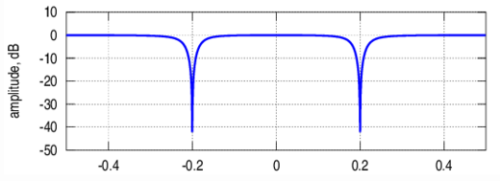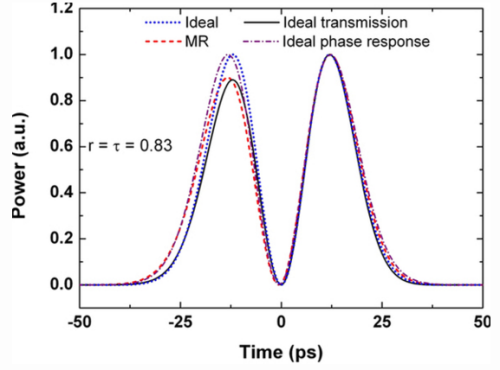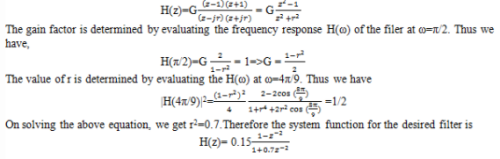Courses

Test: Frequency Selective Filters

10 Questions MCQ Test Digital Signal Processing | Test: Frequency Selective Filters

Description
Attempt Test: Frequency Selective Filters | 10 questions in 10 minutes | Mock test for Electrical Engineering (EE) preparation | Free important questions MCQ to study Digital Signal Processing for Electrical Engineering (EE) Exam | Download free PDF with solutions
QUESTION: 1

If the system has a impulse response as h(n)=Asin(n+1)ω0u(n), then the system is known as Digital frequency synthesizer.

Solution:

Explanation: The given impulse response is h(n)=Asin(n+1)ω0u(n).
According to the above equation, the second order system with complex conjugate poles on the unit circle is a sinusoid and the system is called a digital sinusoidal oscillator or a Digital frequency synthesizer.

QUESTION: 2

The filter with the system function H(z)=z -k is a:

Solution:

Explanation: The system with the system function given as H(z)=z -k is a pure delay system . It has a constant gain for all frequencies and hence called as All pass filter.

QUESTION: 3

A comb filter is a special case of notch filter in which the nulls occur periodically across the frequency band.

Solution:

Explanation: A comb filter can be viewed as a notch filter in which the nulls occur periodically across the frequency band, hence the analogy to an ordinary comb that has periodically spaced teeth.

QUESTION: 4

Which of the following filters have a frequency response as shown below?Solution:

Explanation: The given figure represents the frequency response characteristic of a notch filter with nulls at frequencies at ω0 and ω1.

QUESTION: 5

A digital resonator is a special two pole band pass filter with the pair of complex conjugate poles located near the unit circle.

Solution:

Explanation: The magnitude response of a band pass filter with two complex poles located near the unit circle is as shown below.The filter gas a large magnitude response at the poles and hence it is called as digital resonator.

QUESTION: 6

If the low pass filter described by the difference equation y(n)=0.9y(n-1)+0.1x(n) is converted into a high pass filter, then what is the frequency response of the high pass filter?

Solution:

Explanation: The difference equation for the high pass filter is
y(n)=-0.9y(n-1)+0.1x(n)
and its frequency response is given as
H(ω)= 0.1/(1+0.9e-jω).

QUESTION: 7

If hlp(n) denotes the impulse response of a low pass filter with frequency response Hlp(ω), then what is the frequency response of the high pass filter in terms of Hlp(ω)?

Solution:

Explanation: The impulse response of a high pass filter is simply obtained from the impulse response of the low pass filter by changing the signs of the odd numbered samples in hlp(n). Thus
hhp(n)=(-1)n hlp(n)=(e)n hlp(n)
Thus the frequency response of the high pass filter is obtained as Hlp(ω-π).

QUESTION: 8

What is the system function for a two pole band pass filter that has the centre of its pass band at ω=π/2, zero its frequency response characteristic at ω=0 and at ω=π, and its magnitude response is 1/√2 at ω=4π/9?

Solution:

Explanation: Clearly, the filter must have poles at P1,2=re±jπ/2 and zeros at z=1 and z=-1. Consequently the system function isQUESTION: 9

If the phase ϴ(ω) of the system is linear, then the group delay of the system:

Solution:

Explanation: We know that the group delay of the system with phase ϴ(ω) is defined as
Tg(ω)=(dϴ(ω))/dω
Given the phase is linear=> the group delay of the system is constant.

QUESTION: 10

An ideal filter should have zero gain in their stop band.

Solution:

Explanation: For an ideal filter, in the magnitude response plot at the stop band it should have a sudden fall which means an ideal filter should have a zero gain at stop band.Use Code STAYHOME200 and get INR 200 additional OFF Use Coupon Code

Track your progress, build streaks, highlight & save important lessons and more!

Similar ContentRelated tests